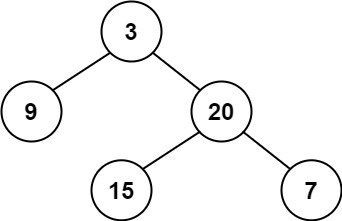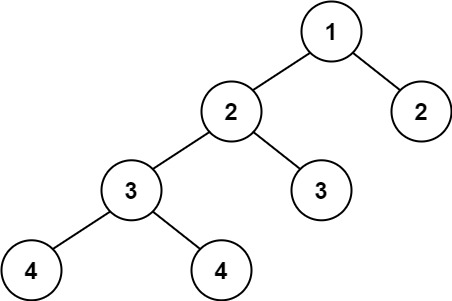# LeetCode: 110. Balanced Binary Tree

## 题目¶

Given a binary tree, determine if it is height-balanced.

For this problem, a height-balanced binary tree is defined as:

a binary tree in which the left and right subtrees of every node differ in height by no more than 1.

Example 1:```Input: root = [3,9,20,null,null,15,7]
Output: true
```

Example 2:```Input: root = [1,2,2,3,3,null,null,4,4]
Output: false
```

Example 3:

```Input: root = []
Output: true
```

Constraints:

• The number of nodes in the tree is in the range [0, 5000].
• -104 <= Node.val <= 104

## 解法¶

```# Definition for a binary tree node.
# class TreeNode:
#     def __init__(self, val=0, left=None, right=None):
#         self.val = val
#         self.left = left
#         self.right = right
class Solution:
def isBalanced(self, root):
if root is None:
return True
left_height = self.max_height(root.left)
right_height = self.max_height(root.right)

return abs(left_height - right_height) <= 1 and self.isBalanced(root.left) and self.isBalanced(root.right)

def max_height(self, root):
if root is None:
return 0
return 1 + max(self.max_height(root.left), self.max_height(root.right))
```